## Tuesday, 25 January 2022

SBI Clerk Day 2 Numerical Ability Online Test 2

1.  Directions(Q.1 to Q.10 ):

What should come in place of the question mark (?) in the following questions ?

28677 ÷ ? = 79 × 11

a
35
b
33
c
1089
d
1225
e
None of theseView Answer
Explanation :

$\frac{28677}{\sqrt{?}}=79 \times 11$

$\Rightarrow \sqrt{?}=\frac{28677}{79 \times 11}=33$

$\therefore ?=33 \times 33=1089$

2.

$\frac{16 \times 12+38}{12 \times 8-25}=?$

a
$2 \frac{1}{2}$
b
$3 \frac{7}{71}$
c
$2 \frac{11}{70}$
d
$4 \frac{35}{142}$
e
None of theseView Answer
Explanation :

$?=\frac{192+38}{96-25}$

$=\frac{230}{71}=3 \frac{17}{71}$

3.

2376 ÷ 32= ?

a
67
b
64.5
c
74.25
d
0.69
e
None of theseView Answer
Explanation :

$?=\frac{2376}{32}=74.25$

4.

$7 \frac{1}{6}$ of $186+565=2100-?$

a
172
b
202
c
222
d
182
e
None of theseView Answer
Explanation :

$186 \times \frac{43}{6}+565=2100-?$

Ÿ 1333 + 565 = 2100 – ?

Ÿ 1898 = 2100 – ?

Ÿ ? = 2100 – 1898 = 202

5.

12321 = ?

a
91
b
81
c
111
d
121
e
None of theseView Answer
Explanation :

$?=\sqrt{12321}=\sqrt{111 \times 111}$

$=111$

6.

67539 + 43908 = ? + 78902

a
33555
b
32455
c
30125
d
31265
e
None of theseView Answer
Explanation :

? = (67539 + 43908) – 78902

= 111447 – 78902 = 32545

7.

12.6 × 10.2 × 6.5 = ?

a
675.58
b
555.68
c
755.48
d
835.38
e
None of theseView Answer
Explanation :

? = 12.6 × 10.2 × 6.5

= 835.38

8.

12540 ÷ 55 ÷ 6 = ?

a
32
b
44
c
46
d
38
e
None of theseView Answer
Explanation :

$?=\frac{12540}{55 \times 6}=38$

9.

48% of 840 = 36% of ?

a
1320
b
1240
c
1080
d
720
e
None of theseView Answer
Explanation :

$? \times \frac{36}{100}=\frac{840 \times 48}{36}$

$\Rightarrow ?=\frac{840 \times 48}{36}=1120$

10.

45% of 300 + ? = 56% of 750 – 10% of 250

a
60
b
67600
c
130
d
260
e
None of theseView Answer
Explanation :

$\frac{300 \times 45}{100}+\sqrt{?}$

$=\frac{750 \times 56}{100}-\frac{250 \times 10}{100}$

$\Rightarrow 135+\sqrt{?}=420-25=395$

$\Rightarrow \sqrt{?}=395-135=260$

$\Rightarrow ?=260 \times 260=67600$

11.  Directions(Q.11 to Q.15 ):

What will come in the place of the question mark (?) in the following number series?

9 11 16 26 ? 69

a
40
b
43
c
41
d
42
e
None of these.View Answer
Explanation :
The pattern is : 9 + 2 (= 12 + 1) = 11 11 + 5 (= 22 + 1) = 16 16 + 10 (= 32 + 1) = 26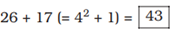43 + 26 (= 52 + 1) = 69

12.

6 3 3 6 24 ?

a
192
b
191
c
190
d
189
e
None of these.View Answer
Explanation :
The pattern is : $6 \times \frac{1}{2}=3$ 3 × 1 = 3 3 × 2 = 6 6 × 4 = 24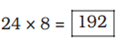13.

21 24 32 45 63 ?

a
86
b
84
c
85
d
82
e
None of these.View Answer
Explanation :
The pattern is : 21 + 3 = 24 24 + 8 (= 3 + 5) = 32 32 + 13 (= 8 + 5) = 45 45 + 18 (= 13 + 5) = 63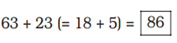14.

3 4 10 33 136 ? 4116

a
700
b
695
c
685
d
590
e
None of these.View Answer
Explanation :
The pattern is : 3 × 1 + 1 = 3 + 1 = 4 4 × 2 + 2 = 8 + 2 = 10 10 × 3 + 3 = 30 + 3 = 33 33 × 4 + 4 = 132 + 4 = 136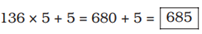685 × 6 + 6 = 4110 + 6 = 4116

15.

7 10 16 28 ? 100

a
50
b
53
c
49
d
51
e
52View Answer
Explanation :
The pattern is : 7 + 3 = 10 10 + 6 (= 2 × 3) = 16 16 + 12 (= 2 × 6) = 28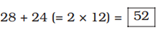52 + 48 (= 2 × 24) = 100

16.  Directions(Q.16 to Q.35 ):

Study the table and answer the given questions.

Marks (out of 100) of 5 students A, B, C, D and E in class tests on different days of a week.

The difference between 67% of a number and 43% of the same number is 912. What is 19% of that number ?

a
722
b
608
c
684
d
798
e
None of theseView Answer
Explanation :

Let the number be x .

According to the question,

(67 – 43)% of x = 912

$\Rightarrow x \times \frac{24}{100}=912$

$\Rightarrow x=\frac{912 \times 100}{24}=3800$

$\therefore 19 \%$ of $3800=\frac{3800 \times 19}{100}$

$=722$

17.

The owner of a computer shop charges his customer 13% more than the cost price. If a customer paid Rs. 15, 933 for a computer, then what was the cost price of the computer ?

a
Rs. 18,200
b
Rs. 12,700
c
Rs. 16,500
d
Rs. 14,100
e
None of theseView Answer
Explanation :

Cost price of computer

$=$ Rs. $\left(\frac{100}{113} \times 15933\right)=$ Rs. 14100

18.

The cost of 12 note-books and 16 pens is Rs. 852. What is the cost of 9 note-books and 12 pens?

a
Rs. 639
b
Cannot be determined
c
Rs. 567
d
Rs. 743
e
None of theseView Answer
Explanation :

Let the CP of 1 note book

= Rs. x and CP of 1 pen = Rs. y.

According to the question,

12x + 16y = 852

Dividing both sides by 4,

3x + 4y = 213

Ÿ 3(3x + 4y) = 3 × 213

Ÿ 9x + 12y = 639

19.

If an amount of Rs. 85,470 is distributed equally amongst 35 children, how much amount would each child get ?

a
Rs. 2,552
b
Rs. 2,542
c
Rs. 2,452
d
Rs. 2,442
e
None of theseView Answer
Explanation :

$=$ Rs. $\left(\frac{85470}{35}\right)=$ Rs. 2442

20.

A sum of money is divided among A, B, C and D in the ratio of 3 : 7 : 9 : 13 respectively. If the share of B is Rs. 4,872, then what is the total amount of money of A and C together ?

a
Rs. 7,456
b
Rs. 9,784
c
Rs. 6,998
d
Rs. 8,352
e
None of theseView Answer
Explanation :

Let the total amount = Rs. x.

Sum of the ratios

= 3 + 7 + 9 + 13 = 32

B’s share = Rs. 4872

$\Rightarrow x \times \frac{7}{32}=4872$

$\Rightarrow x=\frac{4872 \times 32}{7}$

= Rs. 22272

? Amount received by A and C

$=$ Rs. $\left(\frac{12}{32} \times 22272\right)$

= Rs. 8352.

21.

The average age of a man and his son is 18 years. The ratio of their ages is 5 : 1 respectively. What will be the ratio of their ages after 6 years ?

a
10 : 3
b
5 : 2
c
3 : 1
d
4 : 3
e
None of these.View Answer
Explanation :

Let the present age of the man = 5x years and that of son

= x years.

According to the question,

5x + x = 18 × 2

$\Rightarrow 6 x=36 \Rightarrow x=\frac{36}{6}=6$

:Man’s present age = 5 × 6

= 30 years

Son’s present age = 6 years

:Required ratio after 6 years

= 36 : 12 = 3 : 1

22.

In a class of 40 students and 5 teachers, each student got sweets that are 15% of the total number of students and each teacher got sweets that are 20% of the total number of students. How many sweets were there ?

a
260
b
240
c
360
d
320
e
None of these.View Answer
Explanation :

Number of sweets given to

all students

$=40 \times 40 \times \frac{15}{100}=240$

Number of sweets given to all

teachers $=5 \times 40 \times \frac{20}{100}$

$=40$

Number of all sweets

= 240 + 40 = 280

23.

A single person takes 6 minutes to fill a bottle. If from 11 a.m. to 12.30 p.m. 1845 bottles are to be filled, how many persons should be employed on this job ?

a
121
b
135
c
139
d
127
e
None of these.View Answer
Explanation :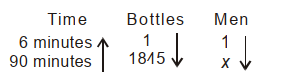where x = number of men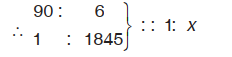Ÿ 90 × x = 6 × 1845

$\Rightarrow x=\frac{6 \times 1845}{90}=123$

24.

In an examination it is required to get 310 of the aggregate marks to pass. A student gets 28% marks and is declared failed by 93 marks. What are the maximum aggregate marks a student can get ?

a
765
b
Cannot be determined
c
775
d
685
e
None of theseView Answer
Explanation :

28% of maximum marks

= 310 – 93 = 217

Ÿ Maximum aggregate marks

$=\frac{217 \times 100}{28}=775$

25.

What is the difference between total score of B on Monday, Wednesday and Thursday together and the total score of E on Tuesday, Wednesday and Friday together?

a
20
b
14
c
12
d
10
e
None of theseView Answer
Explanation :

Total marks obtained by B on Monday, Wednesday and Thursday = 65 + 82 + 72 = 219 Total marks obtained by E on Tuesday, Wednesday and Friday = 72 + 59 + 74 = 205

Required difference = 219 – 205 = 14

26.

If (96)2 is added to the square of a number, the answer so obtained is 16441. What is the number ?

a
91
b
89
c
73
d
67
e
None of these.View Answer
Explanation :

Let the number be x.

According to the question,

x2 + (96)2 = 16441

Ÿ x2 + 9216 = 16441

Ÿ x2 = 16441 – 9216 = 7225

$\Rightarrow x=\sqrt{7225}=85$

27.

What is the respective ratio of total marks scored by C on Tuesday and Wednesday together to the total marks scored by D on the same days together?

a
28 : 23
b
23 : 28
c
29 : 23
d
23 : 29
e
None of theseView Answer
Explanation :

Required ratio

= (70 + 75) : (51 + 64)

= 145 : 115

= 29 : 23

28.

Marks of E on Monday are what per cent more than marks of B on Thursday?

a
15%
b
20%
c
25%
d
$16 \frac{2}{3} \%$
e
None of theseView Answer
Explanation :

Required percentage

$=\left(\frac{84-72}{72}\right) \times 100$

$=\frac{1200}{72}=\frac{50}{3}=16 \frac{2}{3} \%$

29.

What is the compound interest accrued on an amount of Rs. 15,000, at the rate of 11.p.c.p.a. at the end of 3 years ?

a
Rs. 3,575.565
b
Rs. 4,935.895
c
Rs. 5,825.345
d
Rs. 5,514.465
e
None of theseView Answer
Explanation :

C.I. $=\mathrm{P}\left[\left(1+\frac{\mathrm{R}}{100}\right)^{\mathrm{T}}-1\right]$

$=15000\left[\left(1+\frac{11}{100}\right)^{3}-1\right]$

$=15000\left[(1.11)^{3}-1\right]$

= 15000 (1.367631 –1)

= 15000 × 0.367631

= Rs. 5514.465

30.

What is the average marks scored by C on the given days?

a
60
b
62
c
64
d
52
e
None of these.View Answer
Explanation :

Average marks of C

$=\frac{36+70+75+62+77}{5}$

$=\frac{320}{5}=64$

31.

Which number should replace both question marks (?) in the following equation ? $\frac{?}{176}=\frac{44}{?}$

a
92
b
76
c
132
d
88
e
None of these.View Answer
Explanation :

(?)2 = 176 × 44 = 44 × 4 × 44

? = 44 × 2 = 88

32.

One-fifth of a number is 48. What will be 62% of that number ?

a
164.66
b
178.22
c
148.8
d
182.44
e
None of theseView Answer
Explanation :

Let the number be x.

According to the question,

$\frac{x}{5}=48 \Rightarrow x=5 \times 48=240$

$=\frac{62 \% \text { of } 240}{100}=148.8$

33.

42 per cent of first number is 56 per cent of the second number. What is the respective ratio of the first number to the second number ?

a
Cannot be determined
b
4 : 3
c
31 : 42
d
4 : 5
e
None of theseView Answer
Explanation :

Let the first number be x and the second number be y.

According to the question,

\begin{aligned} & x \times \frac{42}{100}=y \times \frac{56}{100} \\ \Rightarrow & \frac{x}{y}=\frac{56}{42}=\frac{4}{3} \end{aligned}

34.

Mr. Vijay deposits an amount of Rs. 45,500 to obtain a simple interest at the rate of 11 p.c.p.a for 3 years. What total amount will Mr. Vijay get at the end of 3 years?

a
Rs. 62,015
b
Rs. 65,515
c
Rs. 60,015
d
Rs. 60,515
e
None of theseView Answer
Explanation :

S.I. $=\frac{\text { Principal } \times \text { Time } \times \text { Rate }}{100}$

$=\frac{45500 \times 3 \times 111}{100}=$ Rs. 15015

Required amount

= Rs. (45500 + 15015) = Rs. 60515

35.

Total marks scored by E on all days together are how much more than total marks scored by A on all days together?

a
55
b
45
c
43
d
65
e
None of these.View Answer
Explanation :

Total marks of A = 74 + 69

+ 49 + 55 + 67 = 314

Total marks of E = 84 + 72 +

59 + 68 + 74 = 357

Required per cent

$=\left(\frac{357-314}{314}\right) \times 100$

$=\frac{4300}{314} \approx 14 \%$